# AP物理1选择题真题讲解第一期

AP物理1选择题（力，动量与动能）

AP Physics  Practice Exam 1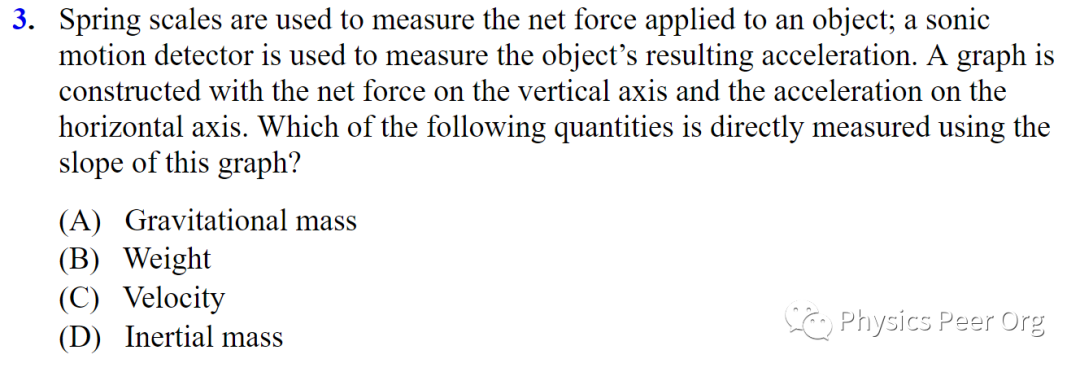D—The relevant equation connecting force and mass is F = ma. The slope is the vertical axis divided by the horizontal axis, or F/a = m. So the slope is mass.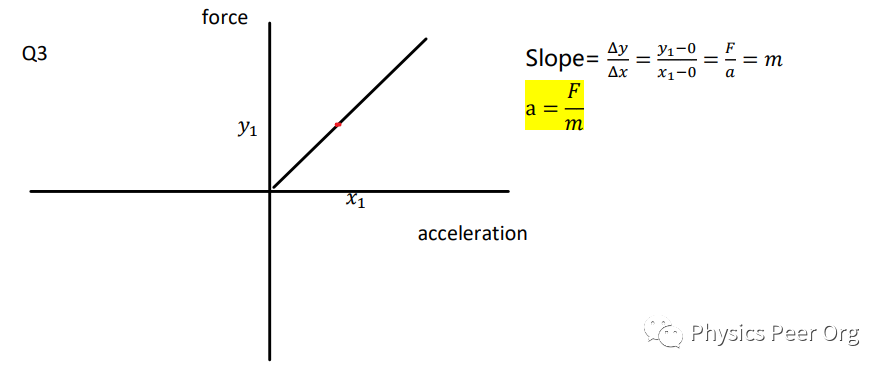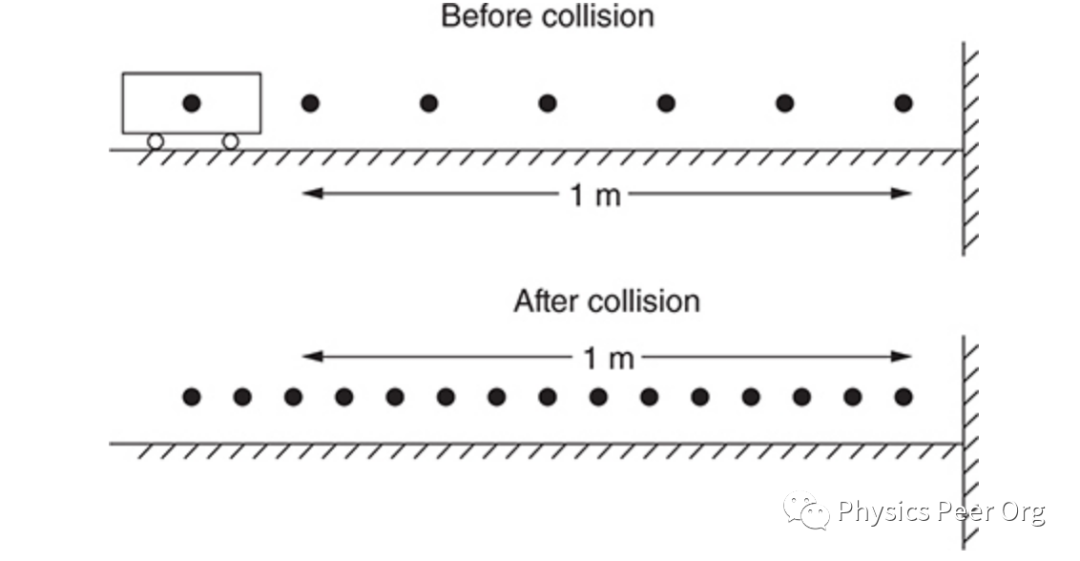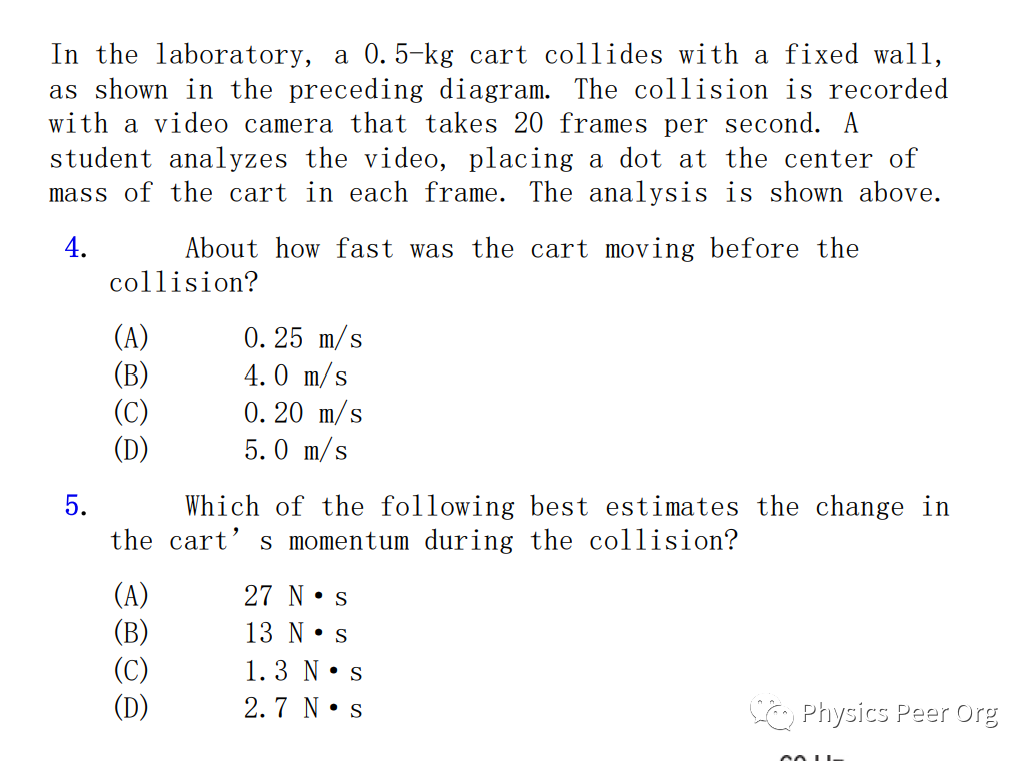4. B—The dots divide the 1-meter distance into five parts. In the time between dots, the cart travels  0.2 m. The time between dots is 0.05 s. At constant speed, the speed is given by distance/time: 0.20 m/0.05 s = 4 m/s.

5. D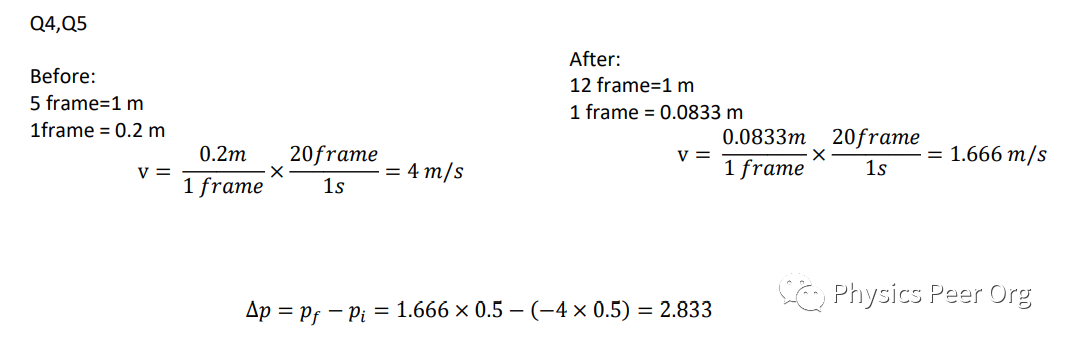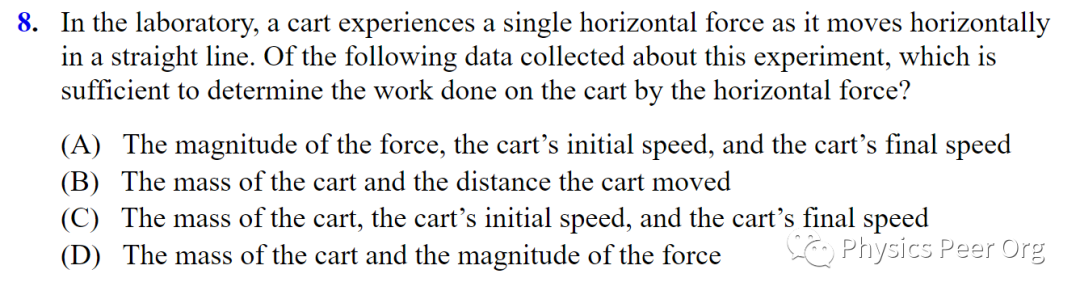C—The kinetic energy (Image) change can be determined by knowing the mass and the speed change.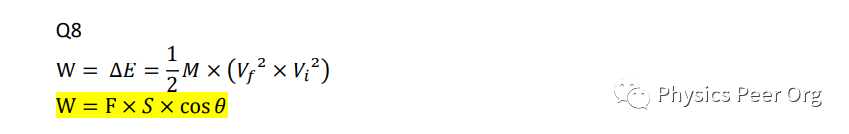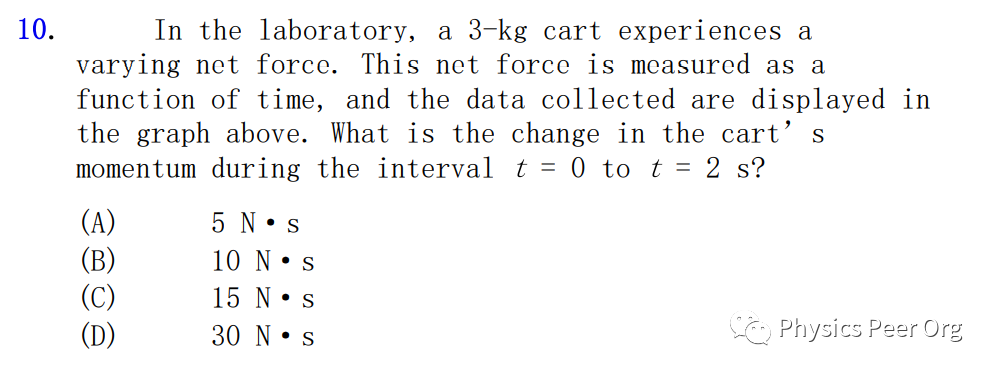A—On this graph, the multiplication of the axes means to take the area under the graph. The area of a triangle is ½(base)(height). That’s ½(5 N)(2 s) = 5 N·s.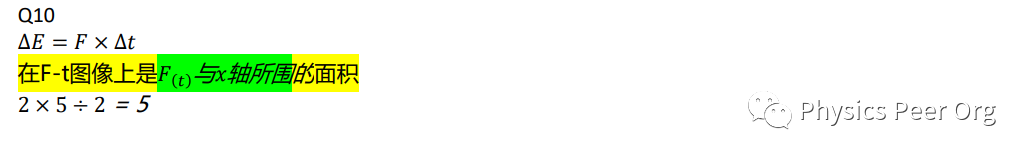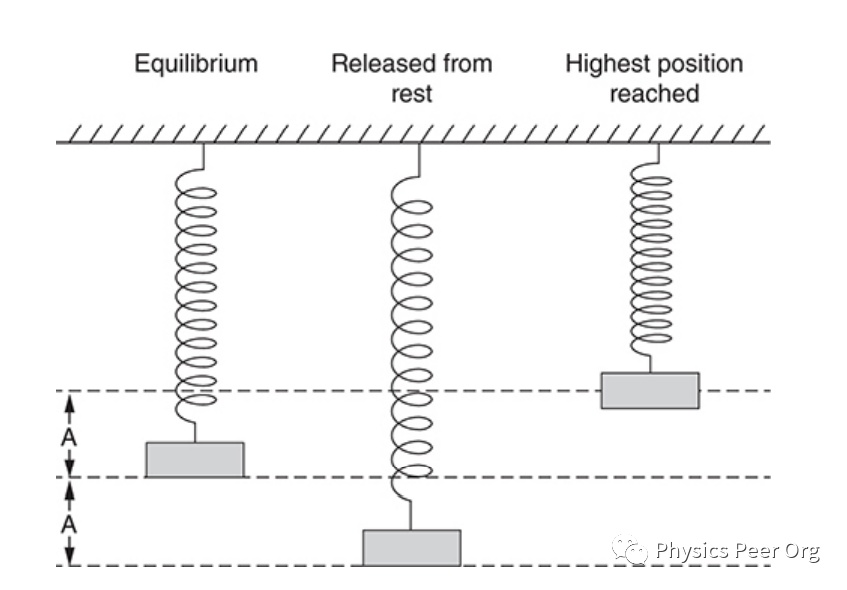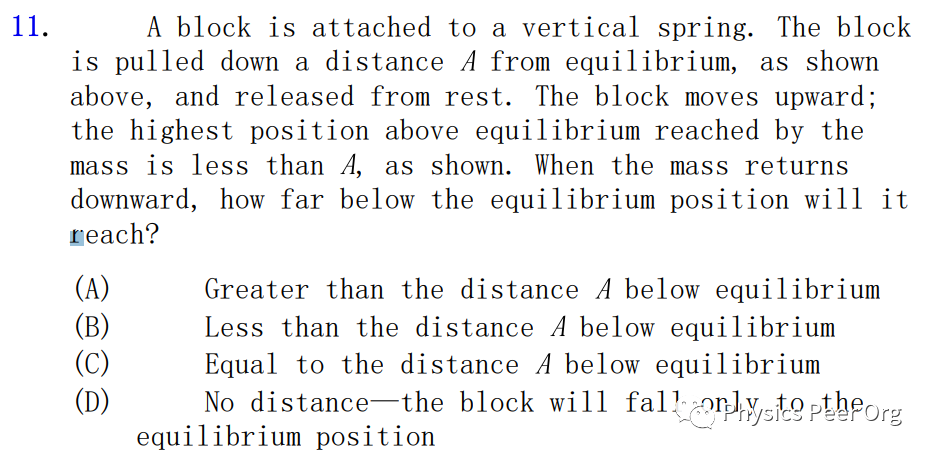B—If friction and air resistance are negligible, a mass on a spring oscillates about the equilibrium position, reaching the same maximum distance above and below. In this case, since the mass doesn’t get all the way to position A at the top, mechanical energy was lost (to friction or air resistance or some nonconservative force). Thus, without some external energy input, the mass won’t reach its maximum position at the bottom, either—at the bottom, it will have no kinetic energy, so all the energy will be potential, and we’ve already established that some total mechanical energy was lost.（别看解析这么长，其实就是因为它要克服摩擦力和空气阻力做功，造成了能量损失）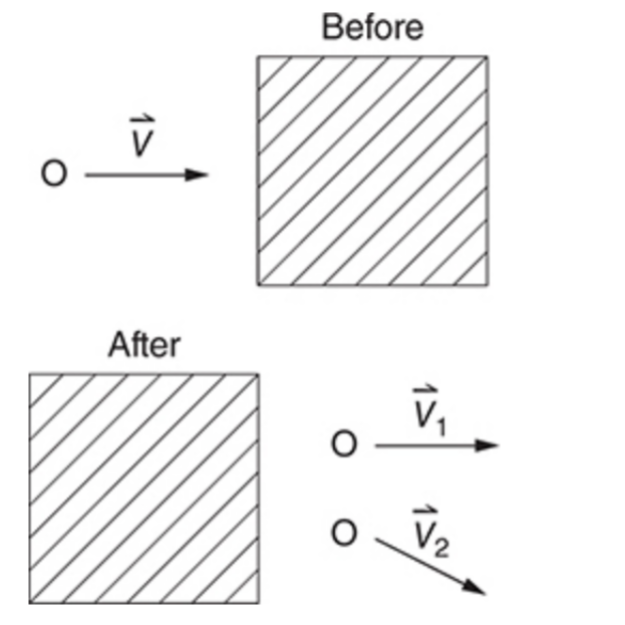### AP物理1选择题真题讲解第二期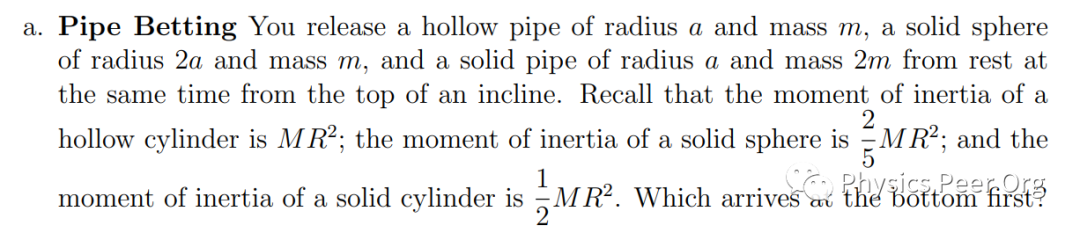5. ### AP美国历史预备课程辅导商务合作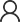在线咨询